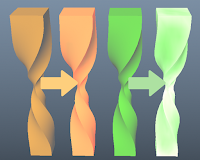## Wednesday, November 8, 2017### Simple sub surface scattering in maya

I think PyMel is definitely the way the maya python API should have been designed from the start but it also takes the user away from the architecture maya is built on. I love using Pymel for various reasons but in order to become a better tech artist I am trying to force myself to using OpenMaya more often.

I used to work with a tech artist before that really contributed a huge amount to me wanting to learn scripting and he made script in 3dmax to bake mesh thickness down to vertex colors. I thought this could be a fun little challenge and here is what I came up with.The idea is to do raycasts from each vertex into random directions (the amount is specified by the samples) and to get the distance  where it has hit the mesh again. Then normalise that based on the average distance and remap it to the vertex colors.

Here you can see the raw output with different amount of samples. Depending on the amount of samples and verts this can take some time. As you can see, good results usually start showing at 256+ unless you are going for an N64 lava level type of look 😃

After calculating the vertex colors I am also doing a gaussian blur pass which makes it look quite nice.

Here is another screen shot with the raw data on the left followed by 1x2x2, 2x2x2, 3x2x2 and a 4x2x2  gaussian blur. This was calculated with 128 samples.

Here are the results on a character, original on the left and the calculated with 2x2x2 blur in different colors.

When projected on to a texture this can also be used as a pretty decent start for a SSS map. Here its cycling through no SSS, light SSS and exaggerated SSS for demonstration purposes

See below for the code

```from random import uniform
import math
import maya.OpenMaya as om
import pymel.core as pm

data = {}
samples = 256

sel = pm.selected()
shape = sel.getShape()

total_progress = len(pm.selected().verts)
current_progress = 0.0

window = pm.window(title="Baking...",minimizeButton=False,maximizeButton=False)
pm.columnLayout()
progressbar = pm.progressBar(maxValue=total_progress*samples, width=300)
pm.showWindow( window )

def remap_val(value, old_min, old_max, new_min, new_max):
old_range = (old_max - old_min)
new_range = (new_max - new_min)
return (((value - old_min) * new_range) / old_range) + new_min

def gaussian(x, mu, sig):
return math.exp(-math.pow(x - mu, 2.) / (2 * math.pow(sig, 2.)))

inMesh = om.MFnMesh(shape.__apimdagpath__())
inMeshMPointArray = om.MPointArray()
inMeshNormalsArray = om.MFloatVectorArray()

inMesh.getPoints(inMeshMPointArray, om.MSpace.kWorld)
inMesh.getNormals(inMeshNormalsArray, om.MSpace.kWorld)
accelParams = inMesh.autoUniformGridParams()

for i in range(inMeshMPointArray.length()):
raySrc = om.MFloatPoint(inMeshMPointArray[i], inMeshMPointArray[i], inMeshMPointArray[i])
val = 0.0
for s in range(samples):
rayDir = om.MFloatVector(uniform(-1.0, 1.0), uniform(-1.0, 1.0), uniform(-1.0, 1.0))
hitPoints = om.MFloatPointArray()
rayHit = inMesh.allIntersections(raySrc, rayDir, None, None, False, om.MSpace.kWorld, 10000000, True,
accelParams, True, hitPoints, None, None, None, None, None, 0.000001)
if (rayHit and hitPoints.length() >= 1):
hitPoint = om.MPoint(hitPoints.x, hitPoints.y, hitPoints.z)
closestPoint = om.MPoint()
inMesh.getClosestPoint(hitPoint, closestPoint, om.MSpace.kWorld)
val += om.MVector(closestPoint-om.MPoint(raySrc)).length() / samples
data[i] = val
current_progress += 1
pm.progressBar(progressbar, edit=True, step=current_progress)

pm.deleteUI( window, window=True )

def assign_colors():
_min, _max = min(data.values()), max(data.values())

eps = sum(data.values()) / (len(data) ** 2 - len(data))

values = []

for v in data:
if data[v] >= 0.0000000001:
normalized_val = remap_val(data[v], _min, _max, 1.0, 0.0)
else:
normalized_val = 0.0
values.append(normalized_val)

# Assign vertex colors
colors = om.MColorArray()
for i in range(inMeshMPointArray.length()):
colors.append(om.MColor())

numColors = colors.length()

colorComp = om.MFnSingleIndexedComponent()
fullComponent = colorComp.create( om.MFn.kMeshVertComponent )
colorComp.setCompleteData( numColors )

vertexIdx = om.MIntArray()
colorComp.getElements(vertexIdx)

for v in range(numColors):
colors[v].r = values[v]
colors[v].g = values[v]
colors[v].b = values[v]

inMesh.setVertexColors(colors, vertexIdx, None)

def blur():
colors = []
window2 = pm.window(title="Blurring colors...")
pm.columnLayout()
progressbar2 = pm.progressBar(maxValue=len(shape.verts)*2, width=300)
pm.showWindow( window2 )
current_progress = 0

for v in shape.verts:
r, g, b = 0.0, 0.0, 0.0
neighbors = list(v.connectedVertices())
num_neighbors = len(neighbors)
gauss = gaussian(0.0, 1.0,-1.0)
gauss2 = gaussian(-0.5, 1.0,-1.0)
for i in neighbors:
col = i.getColor()
r += col * gauss
g += col * gauss
b += col * gauss

neighbors2 = list(i.connectedVertices())
num_neighbors2 = len(neighbors2)
for ii in neighbors2:
col2 = ii.getColor()
r += col2 * gauss2
g += col2 * gauss2
b += col2 * gauss2

r = (r + v.getColor())
g = (g + v.getColor())
b = (b + v.getColor())

colors.append([r / (num_neighbors+1*num_neighbors2+1), g / (num_neighbors+1*num_neighbors2+1), b / (num_neighbors+1*num_neighbors2+1)])
pm.progressBar(progressbar2, edit=True, step=1)

for v in range(len(colors)):
shape.verts[v].setColor(colors[v])
pm.progressBar(progressbar2, edit=True, step=1)

pm.progressBar(progressbar2, edit=True, endProgress=True)
pm.deleteUI( window2, window=True )

assign_colors()
blur()

pm.setAttr("%s.displayColors" % sel.name(), 1)

```